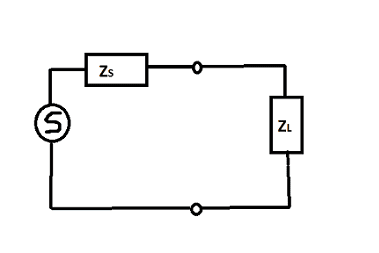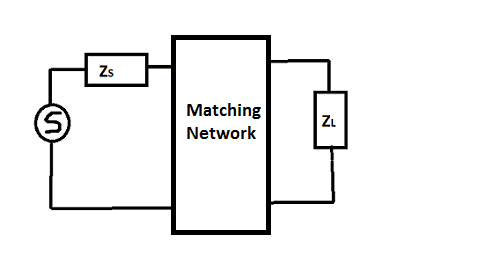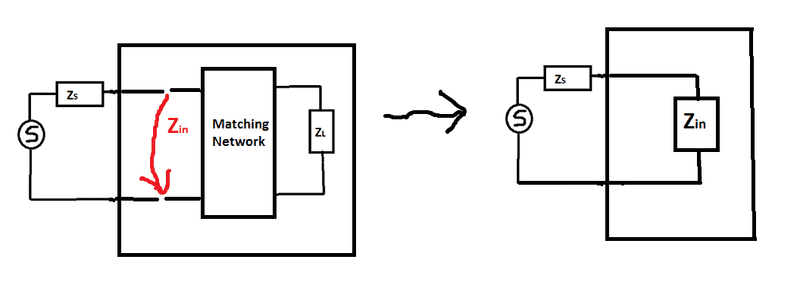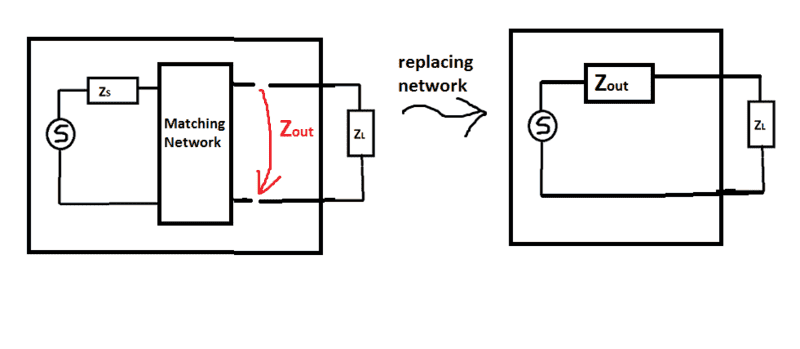# Impedance Matching: The mathematical Conditions for Matching Network

• The Tortoise-Man
In summary, the conversation discusses the concept of impedance matching in a network, where a matching network is placed between a source and load with different impedances in order to achieve maximum power transfer. The discussion also addresses the question of which conditions need to be satisfied for impedance matching, including the use of auxiliary impedances and whether both conditions of ##Z^*_s= Z_{in}## and ##Z_{out}= Z^*_L## need to be satisfied or if one is redundant. The conversation is temporarily closed as a potential repost of previous threads.

#### The Tortoise-Man

Assume we have a network consisting of a source with impedance ##Z_S## and load with impedance ##Z_L## and
we want to perform impedance matching on them in order to obtain the maximum power transfer:Note that in practice there may occur sitations where causes more harm than profit (see eg Baluncore's example here: https://www.physicsforums.com/threa...g-when-the-transmitter-line-and-load.1009889/ ; see post #2)

But the motivation of this question has pure conceptional nature; ie I'm not asking here IF it should be done but HOW it should be correctly done, if one has to do it.

In general, ##Z_S \neq Z_L^*##. So principally, what one do in order to match impedances, one places a matching network between source and load (the components which the matching network contains depend on concrete problem):Indeed, the matching network can consist of resistive components, it might be a L- or T-network and and and... ) and one tries to adjust the parameters of the components of the matching network to satisfy certain matching conditions; see below.

Now having implemented the matching network in our circuit in order to impose the right mathematical conditions we introduce following two auxilary impedances: the input impedance ##Z_{in}## and output impedance ##Z_{out}## defined as follows:My question is: In order to obtain the impedance matching
for maximal power transfer which conditions should be satisfied?

##Z^*_s= Z_{in}##, ##Z_{out}= Z^*_L## or both simultaneously?

Or even more interesting question is: Assume we succeed in adaping the components within the matching network such that ##Z^*_s= Z_{in}## holds. Is then ##Z_{out}= Z^*_L##
automatically satisfied?

So the question is basically about if it really neccassary to adapt the components within the matching network such that they should satisfy both conditions ##Z^*_s= Z_{in}## AND ##Z_{out}= Z^*_L##, or is one of these conditions really redundant in the sense that it suffice to adjust the matching box only to satisfy ONE of them and the second is the satisfied automatically?

Thread closed temporarily as a potential repost of previous threads. Hopefully I can deal with this before the weekend.

The Tortoise-Man said:
Sorry, you are right, presumably sending a PM to you about how to continue the discussion there would have been a better option. I will keep that in mind.

## 1. What is impedance matching?

Impedance matching is the process of adjusting the impedance of a circuit to match the impedance of another circuit or component in order to maximize power transfer and minimize signal reflections.

## 2. Why is impedance matching important?

Impedance matching is important because it ensures that the maximum amount of power is transferred from one circuit to another, reducing signal loss and distortion. It also helps to prevent damage to sensitive components due to mismatched impedances.

## 3. What are the mathematical conditions for impedance matching?

The mathematical conditions for impedance matching require that the source impedance and the load impedance are equal, and that the impedance of the matching network is purely resistive. This can be achieved using various techniques such as using inductors, capacitors, and resistors in a specific configuration.

## 4. How do I calculate the impedance of a matching network?

The impedance of a matching network can be calculated using the Smith chart, which is a graphical tool that helps to visualize and calculate impedance matching. It can also be calculated using mathematical equations and formulas, depending on the specific circuit and components used in the matching network.

## 5. What are the consequences of not using impedance matching?

Not using impedance matching can result in signal reflections, which can cause distortion and loss of power in the circuit. This can affect the overall performance and reliability of the circuit, and may also lead to damage to sensitive components. In some cases, not using impedance matching can also cause interference with other circuits and devices.